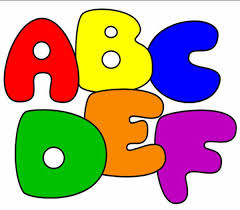# Think Simply..... 3

Level pendingWhat can the next letter be in the series ??

M, W, F, S, T, ??

DETAILS and ASSUMPTIONS :-

For example, the answer might be F even though it is not. The numerical position of F is 6.

The answer is in the form of a variable 'x'. Provide the answer as x - 2.

In the example, the answer is F i.e. the numerical position is 6 THEREFORE the ANSWER IS 6 -2 = 4

×